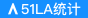# 石材机械 重庆石材机械13562706597

• 价格要求： 电议
• 采购数量：1000
• 包装要求：无要求
• 采购情况：长期有效
• 所在地：山东
• 发布日期：2020-08-20 23:55
• 信息有效期： 发布者未注册，不计时。登录后方可查看联系方式

• 所在地：山东
• 有效期至：长期有效
• 浏览次数：619

r 石材机械r

r

r r

r 济宁市安源机械设备厂专业生产销售各种优质多片锯石机。物美价廉的多片锯石机，多片锯石机采用国际**技术，高效，优质，安全，快捷，安源牌多片锯石机是您的明智之选。多片锯石机客户**信赖；我公司是目前国内生产墙壁切割机品种**全**的厂家。r

r r r r r r r r r r r r r r r r r r r r r r r r
 r r 额定输入功率：45000（W）r r r r 锯片直径：1600-1800（mm）r r r r 加工定制：板材加工r r r r **大锯切深度：700（mm）r r r r 重量：5000（Kg）r r r r 电源类型：交流电源r r r r 型号：HDJ-1600r r r r 规格：HDJ-1600r r r r 适用范围：石材机械r r r r 品牌：安源机械r r r r 电源电压：380Vr r r r 空载转速：980（r/min）r r
r

r 济宁市安源机械设备厂r

r

r 联系人：刘倩r

r

r 手机: 13562706597 r

r

r 电话：0537-2108028r

r

r 传真：0537-2108028r

r

r QQ:  2510618178r

r

r 旺旺：jnanyuan01r

r

r 邮箱:2510618178@qq.comr

r

r 近几年，石材市场对路边石、台阶石、大理石板材的加工效率的需求量大幅度提升。针对这一现况，我厂成功开发出了一种先进的路边石、台阶石、大理石板材的专业加工设备AY-1600(1800)MM型多片锯石机，使生产效率提高了多倍。AY-1600(1800)MM型多片锯石机可同时安装锯片6张（锯片选择薄型为佳，可减少切割阻力）；立柱梁可自动上下移动切割，方便定尺；矿车自动行走。如果给其配备2-3台大锯标胚，那么每个班次下来至少可生产成品600米。这样一来不仅节约了设备的用电量，有节省了人工成本，相应地加工成本**大幅降低，利润也**提高了。所以选择多片锯实在是路边石、台阶石、大理石板材加工的**。r

r r r r r r r r r r r r r r r r r r r r r r r r r r r r r r r r r r r r r r r r r r r r r r r r r
 r r 型号r r r r 锯片直径（mm）r r r r 横向行程（mm）r r r r 升降行程（mm）r r r r 总功率（KW）r r r r 安装锯片r r r r 重量（kg）r r r r AY-1200r r r r 1200r r r r 2100r r r r 1500r r r r 38.5r r r r 8片r r r r 4200r r r r AY-1600r r r r 1600r r r r 2100r r r r 1500r r r r 48.5r r r r 6片r r r r 4800r r r r AY-1800r r r r 1800r r r r 2600r r r r 1500r r r r 58.5r r r r 6片r r r r 6500r r r r AY-2000r r r r 2000r r r r 2600r r r r 1500r r r r 58.5r r r r 4片r r r r 8000r r
r

r 公司主营：地钻 安源地钻 济宁地钻  植树挖坑机 植树钻孔机 挖坑机 刨坑机 电动金刚石链锯 钢筋混凝土开槽机 煤矿防爆开槽机 链条锯 割煤设备 锯煤机设备 挖煤机设备 采煤机设备 矿用链锯 汽油，电动，带土球起苗机 挖树机 刨树机 切墙机、锯墙机 墙壁开槽机 钢筋混凝土墙壁切割机 油锯等 高枝油锯 高位树枝修枝锯 高枝剪,花具,修枝剪 油锯伐木锯 汽油锯 汽油链锯 优质油锯 冰块切割机 手提式冰锯 劈开机 一次成型墙壁开槽机 多功能水电开槽机等r

rr
r

0相关评论

#### 联系方式

(c)2008-2021 www.sdzhst.cn B2B SYSTEM All Rights Reserved